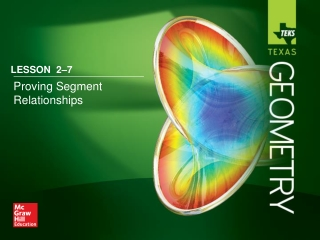DownloadDownload PresentationLESSON 2 –7

# LESSON 2 –7

Download Presentation## LESSON 2 –7

- - - - - - - - - - - - - - - - - - - - - - - - - - - E N D - - - - - - - - - - - - - - - - - - - - - - - - - - -
##### Presentation Transcript

1. LESSON 2–7 Proving Segment Relationships

2. Five-Minute Check (over Lesson 2–6) TEKS Then/Now Postulate 2.8: Ruler Postulate Postulate 2.9: Segment Addition Postulate Example 1: Use the Segment Addition Postulate Theorem 2.2: Properties of Segment Congruence Proof: Transitive Property of Congruence Example 2: Real-World Example: Proof Using Segment Congruence Lesson Menu

3. State the property that justifies the statement. 2(LM + NO) = 2LM + 2NO A. Distributive Property B. Addition Property C. Substitution Property D. Multiplication Property 5-Minute Check 1

4. State the property that justifies the statement.If mR = mS, then mR + mT = mS + mT. A. Distributive Property B. Substitution Property C. Addition Property D. Transitive Property 5-Minute Check 2

5. State the property that justifies the statement.If 2PQ = OQ, then PQ = A. Multiplication Property B. Division Property C. Distributive Property D. Substitution Property 5-Minute Check 3

6. State the property that justifies the statement. mZ = mZ A. Reflexive Property B. Symmetric Property C. Transitive Property D. Substitution Property 5-Minute Check 4

7. State the property that justifies the statement. If BC = CD and CD = EF, then BC = EF. A. Reflexive Property B. Symmetric Property C. Substitution Property D. Transitive Property 5-Minute Check 5

8. Which statement shows an example of the Symmetric Property? A.x = x B. If x = 3, then x + 4 = 7. C. If x = 3, then 3 = x. D. If x = 3 and x = y, then y = 3. 5-Minute Check 6

9. Targeted TEKS G.6(A) Verify theorems about angles formed by the intersection of lines and line segments, including vertical angles, and angles formed by parallel lines cut by a transversal and prove equidistance between the endpoints of a segment and points on its perpendicular bisector and apply these relationships to solve problems. Mathematical Processes G.1(D), G.1(G) TEKS

10. You wrote algebraic and two-column proofs. • Write proofs involving segment addition. • Write proofs involving segment congruence. Then/Now

11. Concept

12. Concept

13. Proof: Statements Reasons ___ ___ AB  CD 1. 1. Given 2. 2. Definition of congruent segments AB = CD 3. 3. Reflexive Property of Equality BC = BC 4. 4. Segment Addition Postulate AB + BC = AC Use the Segment Addition Postulate Example 1

14. Proof: Statements Reasons 5. CD + BC = AC 5. Substitution Property of Equality ___ ___ AC  BD 6. 6. Segment Addition Postulate CD + BC = BD 8. 8. Definition of congruent segments 7. 7. Transitive Property of Equality AC = BD Use the Segment Addition Postulate Example 1

15. Prove the following. Given:AC = ABAB = BXCY = XD Prove:AY = BD Example 1

16. Which reason correctly completes the proof? Proof: Statements Reasons 1. 1. Given AC = AB, AB = BX 2. 2. Transitive Property AC = BX CY = XD 3. 3. Given 4. AC + CY = BX + XD 4. Addition Property 5. 5. ________________ ? AC + CY = AY; BX + XD = BD 6. 6. Substitution AY = BD Example 1

17. A. Addition Property B. Substitution C. Definition of congruent segments D. Segment Addition Postulate Example 1

18. Concept

19. Concept

20. BADGE Jamie is designing a badge for her club. The length of the top edge of the badge is equal to the length of the left edge of the badge. The top edge of the badge is congruent to the right edge of the badge, and the right edge of the badge is congruent to the bottom edge of the badge. Prove that the bottom edge of the badge is congruent to the left edge of the badge. Given: Prove: Proof Using Segment Congruence Example 2

21. Proof: Statements Reasons 1. Given 1. 2. Definition of congruent segments 2. 3. 3. Given ___ YZ 4. Transitive Property 4. 5. Substitution 5. Proof Using Segment Congruence Example 2

22. Prove the following. Given: Prove: Example 2

23. Which choice correctly completes the proof? Proof: Statements Reasons 1. Given 1. 2. Transitive Property 2. 3. 3. Given 4. Transitive Property 4. ? 5. _______________ 5. Example 2

24. A. Substitution B. Symmetric Property C. Segment Addition Postulate D. Reflexive Property Example 2

25. LESSON 2–7 Proving Segment Relationships